# How to extract nth word from text string

This post will guide you how to extract the nth word from a text string in excel. How do I get the nth word from text string in a cell using excel formula. How to write a User Defined Function to extract nth word from text.

## Extract nth word

If you want to extract the nth word from a text string in a single cell, you can create an excel formula based on the TRIM function, the MID function, the SUBSTITUTE function, the REPT function and the LEN function.

You can use the SUBSTITUTE function replaces each space character with a number of space characters returned by the REPT function. The number of space characters should be equal to the overall length of the original text in cell. So you also need to use the LEN function to combine with the REPT function to create those spaces. Then you can use the MID function to extract the nth word and the starting position can get from the formula (N-1)*LEN(B1)+1 , and the num_chars should be equal to the length of the original text.

Assuming that you want to extract the third word from text string in Cell B1, you can write down the following excel formula:

`=TRIM(MID(SUBSTITUTE(B1," ", REPT(" ",LEN(B1))), (3-1)*LEN(B1) +1, LEN(B1)))`

Let’s see how this formula works:

=REPT(” “,LEN(B1))

This formula will return a new text string that contain a number of the space characters and the number of space character is equal to the length of the original text string in Cell B1. Then it goes into the SUBSTITUTE function as its new_text argument.

=SUBSTITUTE(B1,” “, REPT(” “,LEN(B1)))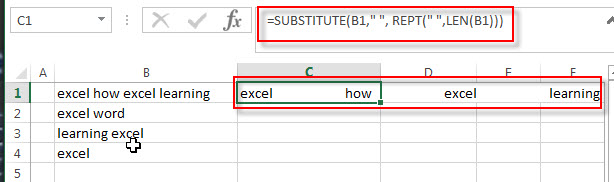This formula replace each space character with new_text that returned by the REPT function. Then it goes into the MID function as its Text argument.

=(3-1)*LEN(B1) +1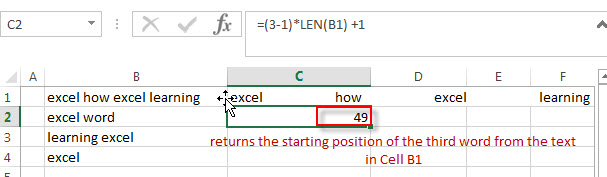This formula returns the starting position of the third word from the text in Cell B1. Then it passed into the MID function as its start_num argument.

=MID(SUBSTITUTE(B1,” “, REPT(” “,LEN(B1))), (3-1)*LEN(B1) +1, LEN(B1))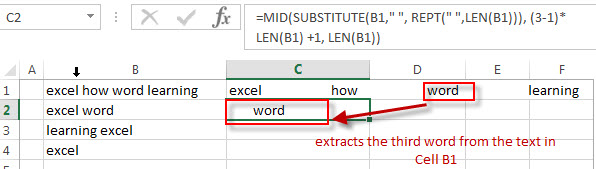The both Text and start_num arguments are returned by the above two formula, so this formula extracts the third word from the text in Cell B1.

=TRIM()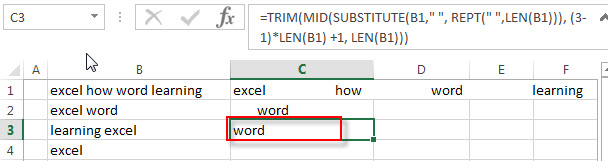The TRIM function removes all extra space characters and just leave only one between words.

## Extract Nth word with User Defined Function

You can also write a User Defined Function to extract the nth word from the text string in a cell in Excel VBA:

1# click on “Visual Basic” command under DEVELOPER Tab.2# then the “Visual Basic Editor” window will appear.

3# click “Insert” ->”Module” to create a new module4# paste the below VBA code into the code window. Then clicking “Save” button.

```Function GetNthWord(Source As String, Position As Integer)
Dim arr() As String
arr = VBA.Split(Source, " ")
xCount = UBound(arr)
If xCount < 1 Or (Position - 1) > xCount Or Position < 0 Then
GetNthWord = ""
Else
GetNthWord = arr(Position - 1)
End If
End Function```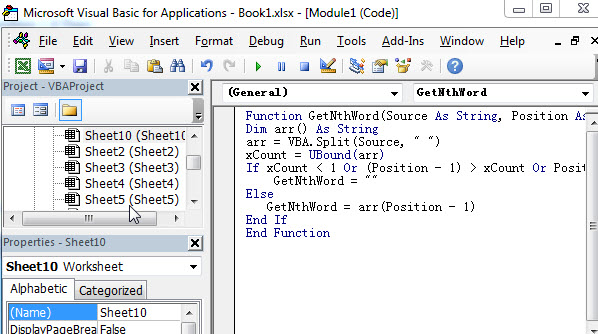5# back to the current worksheet, then enter the below formula in Cell C1: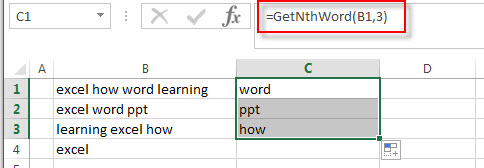### Related Formulas

• count specific words in a cell or a range
If you want to count the number of a specific word in a single cell, you need to use the SUBSTITUTE function to remove all that certain word in text string, then using LEN function to calculate the length of the substring that without that specific word.…
• Get first word from text string
If you want to extract the first word from a text string in a cell, you can use a combination of the IF function, the ISERR function, the LEFT function and the FIND function to create a complex excel formula..…
• Get last word from text string
If you want to get the last word from a text string, you can create an excel formula based on the RIGHT function, the LEN function, the FIND function and the SUBSTITUTE function..…
• Extract word that starting with a specific character
Assuming that you have a text string that contains email address in Cell B1, and if you want to extract word that begins with a specific character “@” sign, you can use a combination with the TRIM function, the LEFT function, the SUBSTITUTE function, the MID function, the FIND function, the LEN function and the REPT function to create an excel formula.…

### Related Functions

• Excel Substitute function
The Excel SUBSTITUTE function replaces a new text string for an old text string in a text string.The syntax of the SUBSTITUTE function is as below:= SUBSTITUTE  (text, old_text, new_text,[instance_num])….
• Excel REPT function
The Excel REPT function repeats a text string a specified number of times.The syntax of the REPT function is as below:= REPT  (text, number_times)…
• Excel TRIM function
The Excel TRIM function removes all spaces from text string except for single spaces between words.  You can use the TRIM function to remove extra spaces between words in a string.The syntax of the TRIM function is as below:= TRIM (text)….
• Excel MID function
The Excel MID function returns a substring from a text string at the position that you specify.The syntax of the MID function is as below:= MID (text, start_num, num_chars)….
• Excel LEN function
The Excel LEN function returns the length of a text string (the number of characters in a text string).The syntax of the LEN function is as below:= LEN(text)…